Even & Odd Numbers Math Video for Kids - K, 1st, & 2nd Grades
1%
It was processed successfully!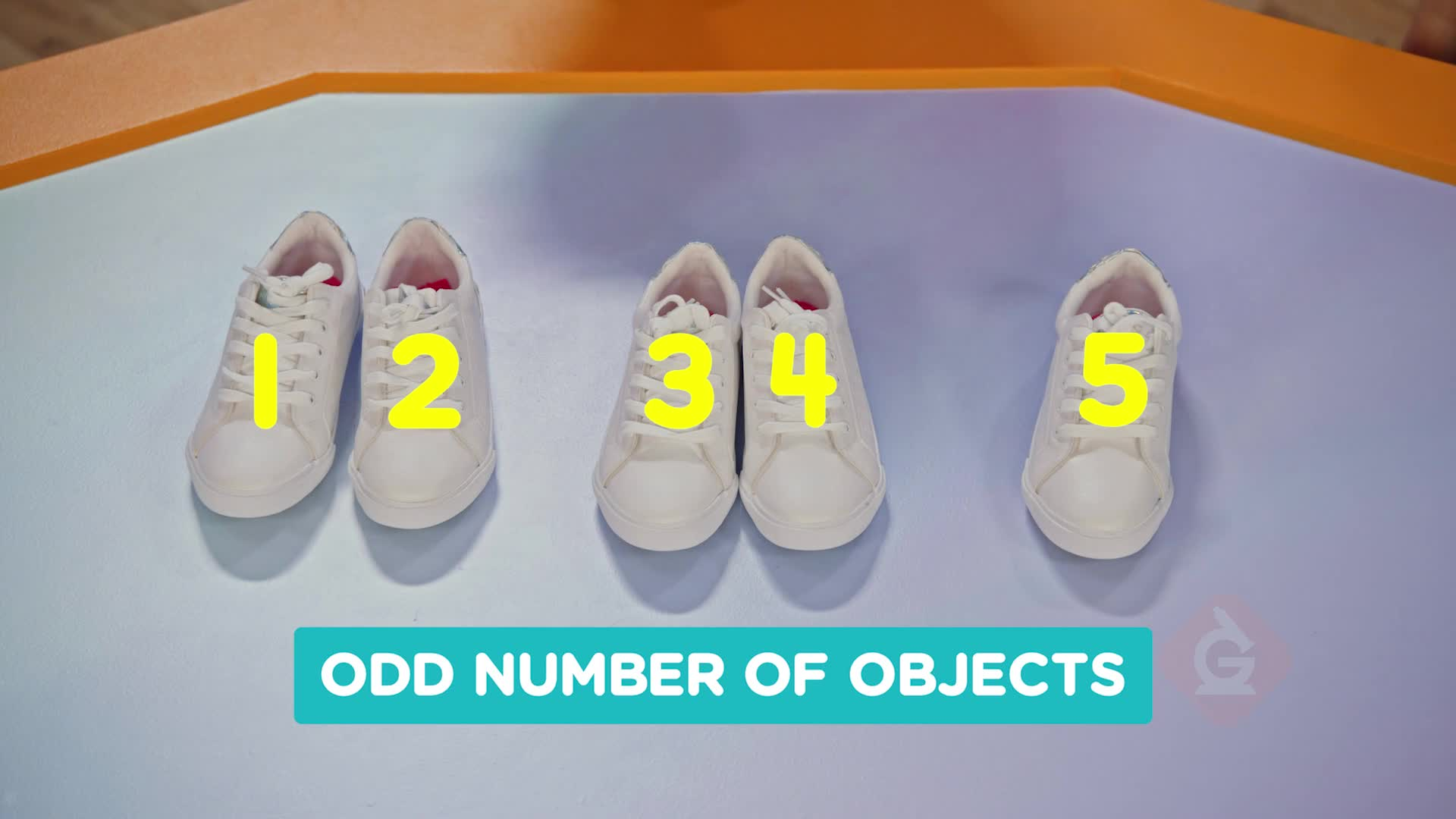WHAT ARE EVEN & ODD NUMBERS?

Numbers can be even or odd. You can tell if a number is even or odd by making pairs, counting by 2s, or looking at the digit in the ones place. Numbers that can be counted by 2s are called even.

To better understand even & odd numbers…

WHAT ARE EVEN & ODD NUMBERS?. Numbers can be even or odd. You can tell if a number is even or odd by making pairs, counting by 2s, or looking at the digit in the ones place. Numbers that can be counted by 2s are called even. To better understand even & odd numbers…

## LET’S BREAK IT DOWN!

### Make pairs of chopsticks.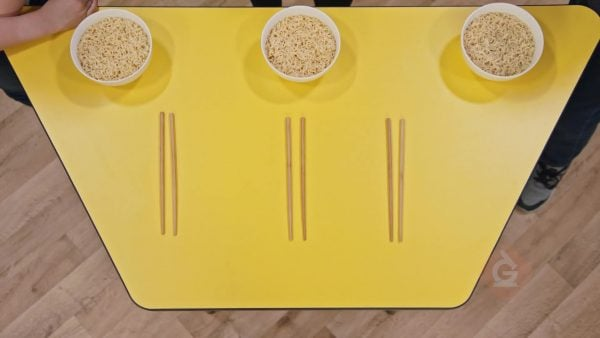You have 6 chopsticks. You can put all 6 chopsticks into groups of 2. 6 is an even number. An even number of objects can be paired in groups of 2 with nothing left over. You can count by 2s to see even numbers: 2, 4, 6, 8. When you count by 2s you add a pair each time.

Make pairs of chopsticks. You have 6 chopsticks. You can put all 6 chopsticks into groups of 2. 6 is an even number. An even number of objects can be paired in groups of 2 with nothing left over. You can count by 2s to see even numbers: 2, 4, 6, 8. When you count by 2s you add a pair each time.

### Make pairs of shoes.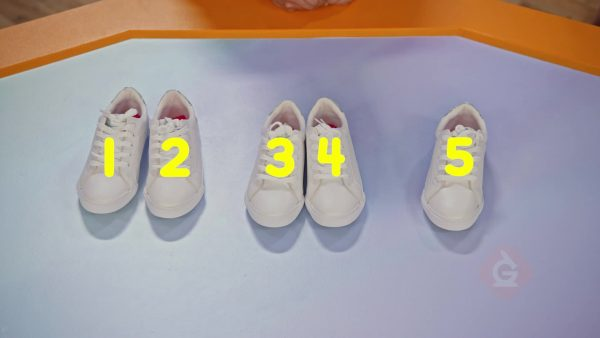You have 6 shoes. The shoes are in pairs. 6 is an even number. If you take 1 shoe away there are now 5 shoes. 1 shoe has no pair. 5 is an odd number. Odd numbers are numbers where one object cannot be paired. 1, 3, 5, 7, and 9 are all odd numbers.

Make pairs of shoes. You have 6 shoes. The shoes are in pairs. 6 is an even number. If you take 1 shoe away there are now 5 shoes. 1 shoe has no pair. 5 is an odd number. Odd numbers are numbers where one object cannot be paired. 1, 3, 5, 7, and 9 are all odd numbers.

### Make pairs of bracelets.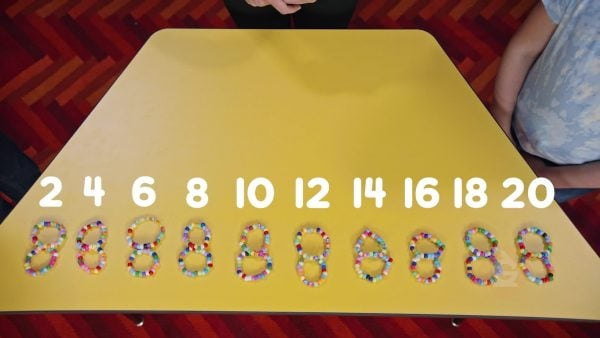You made 20 friendship bracelets. You want to give the bracelets away in pairs. Count the bracelets as you make pairs: 2, 4, 6, 8, 10, 12, 14, 16, 18, 20. 20 is even because 20 objects can be put into pairs.

Make pairs of bracelets. You made 20 friendship bracelets. You want to give the bracelets away in pairs. Count the bracelets as you make pairs: 2, 4, 6, 8, 10, 12, 14, 16, 18, 20. 20 is even because 20 objects can be put into pairs.

### Find the pattern.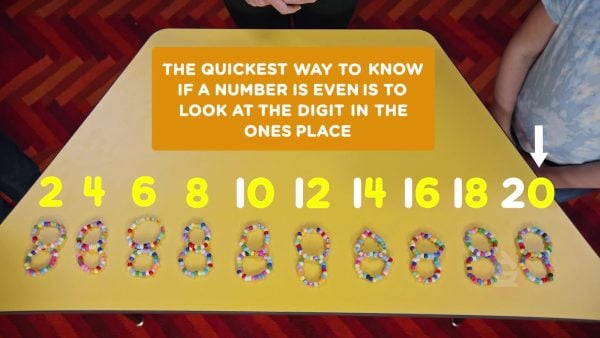Here are some even numbers: 2, 4, 6, 8, 10, 12, 14, 16, 18, 20. Do you see a pattern? The digits in the ones place repeat: 2, 4, 6, 8, 0. If the digit in the ones place is even, then the whole number is even. In 34, 4 is even so 34 is even. In 35, 5 is odd, so 35 is odd.

Find the pattern. Here are some even numbers: 2, 4, 6, 8, 10, 12, 14, 16, 18, 20. Do you see a pattern? The digits in the ones place repeat: 2, 4, 6, 8, 0. If the digit in the ones place is even, then the whole number is even. In 34, 4 is even so 34 is even. In 35, 5 is odd, so 35 is odd.

### Write an equation to show even numbers.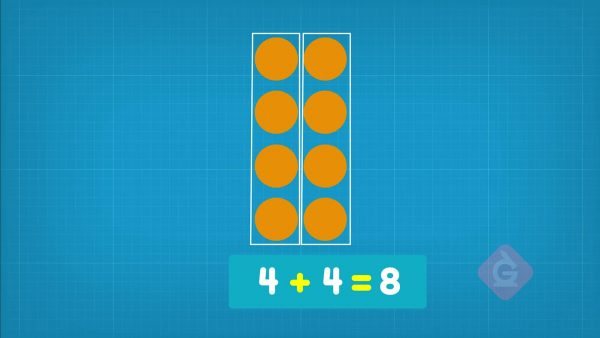Even numbers can be shown in two equal groups. 10 can be put into two groups of 5. 5 + 5 = 10. 16 can be put into two groups of 8. 8 + 8 = 16.

Write an equation to show even numbers. Even numbers can be shown in two equal groups. 10 can be put into two groups of 5. 5 + 5 = 10. 16 can be put into two groups of 8. 8 + 8 = 16.

## EVEN & ODD NUMBERS VOCABULARY

Even number
An even number of objects can be paired in groups of 2 with nothing left over.
Odd number
An odd number of objects cannot be paired in groups of 2 with nothing left over. One of the objects is not paired.
Putting together two or more groups to find a new total.
Sum
Equal Groups
Groups that have the same size or quantity.
Counting while skipping numbers. For example, when you skip count by twos you skip every second number: 2, 4, 6, 8, 10, ….
Numbers, shapes, or objects arranged following a rule or rules.

## EVEN & ODD NUMBERS DISCUSSION QUESTIONS

### How can you tell if a group of objects has an even number of objects?

You could pair them off. If there is an even number of objects, they will go into pairs with nothing left over.

### How can you tell if a group of objects has an odd number of objects?

You could pair them off. If there is an odd number of objects, there will be one object left over.

### [Draw or display 4 objects.] Is this an odd or even number of objects? Why?

Even. I can make two equal groups.

### [Draw or display 13 objects.] Is this an odd or even number of objects? Why?

Odd. When I put the objects into pairs, there is one left over.

### Write an equation that shows 18 put into two equal groups. Is 18 even?

9 + 9 = 18. 18 is even because it can be put into two equal groups.
X

## Success

We’ve sent you an email with instructions how to reset your password.
Ok

## Choose Your Free Trial Period### 7 DaysContinue to Lessons### 90 DaysGet 90 days free by inviting other teachers to try it too.

Share with Teachers

## Get 90 Days Free

### By inviting 4 other teachers to try it too.

4 required

Skip, I will use a 7 days free trial

## Thank You!

Enjoy your free 90 days trial